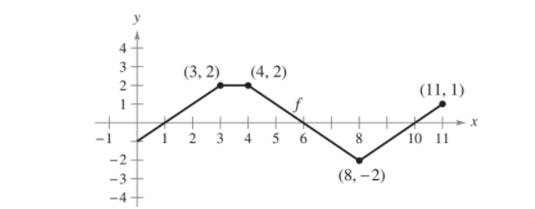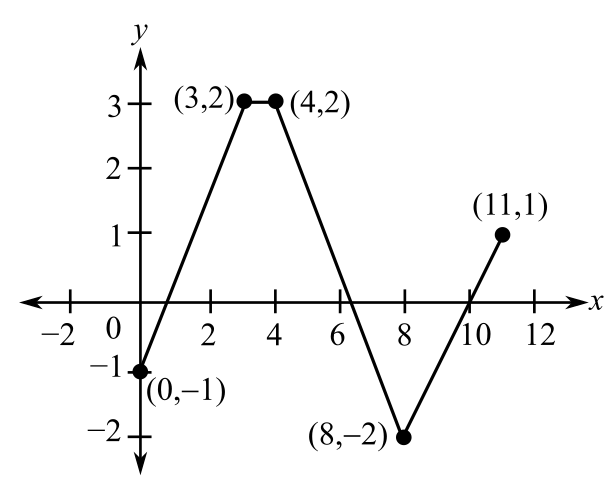Chapter 4.3, Problem 48E

Chapter
Section
Textbook Problem

Think About It The graph of f consists of line segments, as shown in the figure. Evaluate each definite integral by using geometric formulas.(a) ∫ 0 1 − f ( x ) d x (b) ∫ 3 4 3 f ( x ) d x (c) ∫ 0 7 f ( x ) d x (d) ∫ 5 11 f ( x ) d x (e) ∫ 0 11 f ( x ) d x (f) ∫ 4 10 f ( x ) d x

(a)

To determine

To calculate: The integral 01f(x)dx using the given graph;Explanation

Given:

The graph of f consists of line segments is:

Formula used:

If f is continuous and non-negative on the closed interval [a,b] then the area of the region bounded by the graph of f, the x-axis and the vertical lines x=a,x=b that depict the border points is

Area=abf(x)dx

Calculation:

From the given graph, the integral 01f(x)dx is area of triangle with base 1unit and height 1 unit

(b)

To determine

To calculate: The integral 343f(x)dx using the given graph.

(c)

To determine
The integral 07f(x)dx using the given graph.

(d)

To determine

To calculate: The integral 511f(x)dx using the given graph.

(e)

To determine
The integral 011f(x)dx using the given graph.

(f)

To determine
The integral 410f(x)dx using the given graph.

Still sussing out bartleby?

Check out a sample textbook solution.

See a sample solution

The Solution to Your Study Problems

Bartleby provides explanations to thousands of textbook problems written by our experts, many with advanced degrees!

Get Started

Find the derivative of the function. f(t)2t3

Single Variable Calculus: Early Transcendentals, Volume I

In Exercises 4756, solve the given equation for the indicated variable. 4=2x

Finite Mathematics and Applied Calculus (MindTap Course List)

Find the point of intersection of the two straight lines having the equations y =34x + 6 and 3x 2y + 3 = 0.

Applied Calculus for the Managerial, Life, and Social Sciences: A Brief Approach

Evaluate.

Mathematical Applications for the Management, Life, and Social Sciences

Given: BEDACDDA Prove: 12

Elementary Geometry for College Students